# A bottle of wine is 14.5% abv (v/v%). If the wine bottle contains 750 mL of...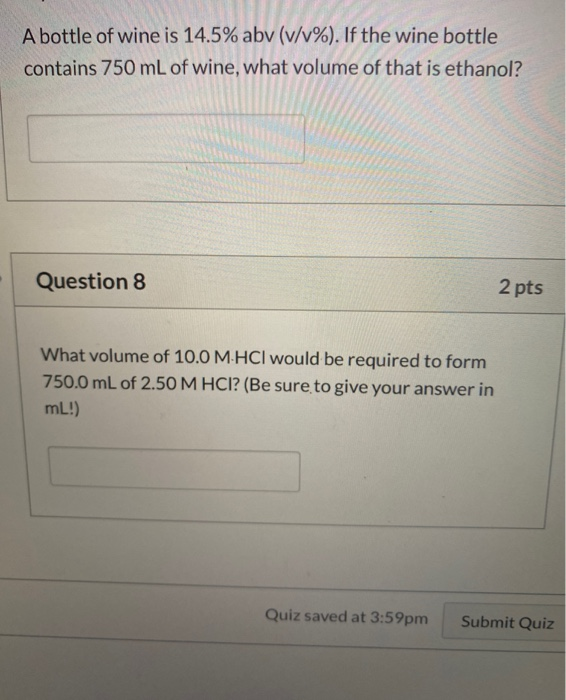A bottle of wine is 14.5% abv (v/v%). If the wine bottle contains 750 mL of wine, what volume of that is ethanol? Question 8 2 pts What volume of 10.0 M.HCl would be required to form 750.0 mL of 2.50 M HCI? (Be sure to give your answer in mL!) Quiz saved at 3:59pm Submit Quiz

This Homework Help Question: "A bottle of wine is 14.5% abv (v/v%). If the wine bottle contains 750 mL of..." No answers yet.

We need 10 more requests to produce the answer to this homework help question. Share with your friends to get the answer faster!

0 /10 have requested the answer to this homework help question.

Once 10 people have made a request, the answer to this question will be available in 1-2 days.
All students who have requested the answer will be notified once they are available.

#### Earn Coin

Coins can be redeemed for fabulous gifts.

Similar Homework Help Questions
• ### principles of chemistry 122 6. A bottle of wine contains 750.0 mL of wine. If the...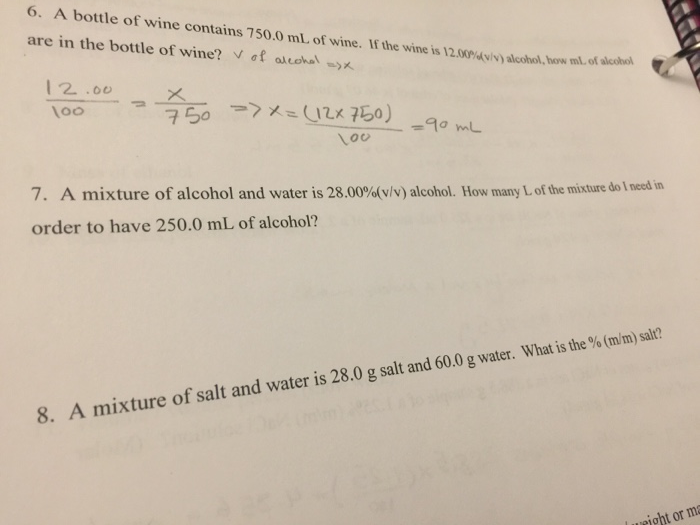principles of chemistry 122 6. A bottle of wine contains 750.0 mL of wine. If the wine is 12.00% are in the bottle of wine? V of alcohol ->X ) alcohol, bow ml of alice 12 .00 100 150 >> x=(12*50) 90 mL loo 7. A mixture of alcohol and water is 28.00%(v/v) alcohol. How many of the mixture do I need in order to have 250.0 mL of alcohol? 8. A mixture of salt and water is 28.0 g...

• ### How much heat must be extracted from 750 mL of white wine to cool it from...

How much heat must be extracted from 750 mL of white wine to cool it from 25 °C to 13 °C, if the wine is 9.6% ethanol by weight? Assume the wine can be treated as an aqueous solution of ethanol, with no other components. The molar heat capacity of water is 75.4 J/mol K and of ethanol is 112.1 J/mol K. [Bonus: Show that this corresponds to a wine that is 12% alcohol by volume, or ABV. Clearly state...

• ### How much heat must be extracted from 750 mL of white wine to cool it from...

How much heat must be extracted from 750 mL of white wine to cool it from 25 °C to 13 °C, if the wine is 9.6% ethanol by weight? Assume the wine can be treated as an aqueous solution of ethanol, with no other components. The molar heat capacity of water is 75.4 J/mol K and of ethanol is 112.1 J/mol K. Also: Show that this corresponds to a wine that is 12% alcohol by volume, or ABV. Clearly state...

• ### A wine-dispensing system uses argon canisters to pressurize and preserve wine in the bottle. An argon...

A wine-dispensing system uses argon canisters to pressurize and preserve wine in the bottle. An argon canister for the system has a volume of 70.2 mL and contains 33.4 g of argon. part a: Assuming ideal gas behavior, what is the pressure in the canister at 318 K ? Express your answer with the appropriate units. part b: When the argon is released from the canister it expands to fill the wine bottle. How many 750.0-mL wine bottles can be...

• ### 4, The 750 mL bottle of Lindeman's Shiraz wine in my kitchen is 7.75 % ethanol...

4, The 750 mL bottle of Lindeman's Shiraz wine in my kitchen is 7.75 % ethanol by volume. The density of ethanol is 0.789 g/mL. What is the molarity of the wine with respect to the solute ethanol? 5. To make a saturated aqueous solution of ammonia, 470 g of ammonia are dissolved with one liter of water. The density of the resulting solution is 0.89 g/mL. What is the molarity of the solution? Hint: Think about the two quantities...

• ### Question 15 1 pts A bottle of cold medicine contains 25% (v/v) ethyl alcohol (ethanol) in...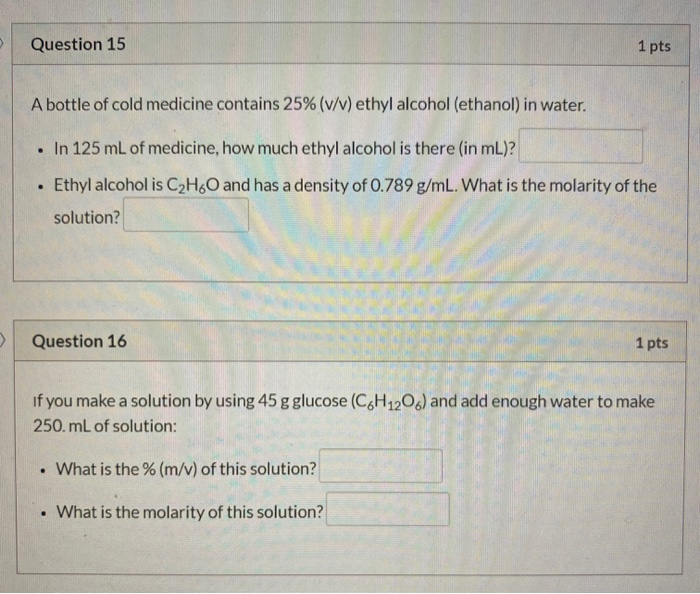Question 15 1 pts A bottle of cold medicine contains 25% (v/v) ethyl alcohol (ethanol) in water. . In 125 mL of medicine, how much ethyl alcohol is there (in mL)? Ethyl alcohol is C2H60 and has a density of 0.789 g/mL. What is the molarity of the solution? . Question 16 1 pts If you make a solution by using 45 g glucose (C6H12O) and add enough water to make 250.mL of solution: . What is the % (m/v)...

• ### A mouthwash contains 22.5% (v/v) alcohol. If the bottle of mouthwash contains 355 mL, what is the volume, in milliliters...

A mouthwash contains 22.5% (v/v) alcohol. If the bottle of mouthwash contains 355 mL, what is the volume, in milliliters , of alcohol? Please solve in simple steps in order for me to understand :) Thank you!

• ### 16) Ethanol, CHsO, is produced by the fermentation of sugars in wine and beer. Given that...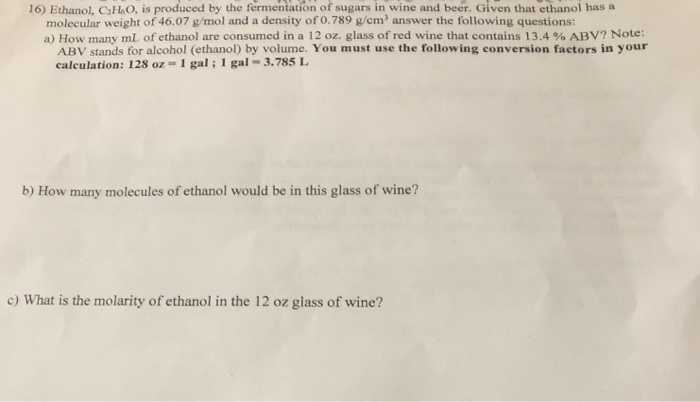16) Ethanol, CHsO, is produced by the fermentation of sugars in wine and beer. Given that ethanol has a molecular weight of 46.07 g/mol and a density of 0.789 g/em answer the following questions: a) How many mL of ethanol are consumed in a 12 oz. glass of red wine that contains 13.4 % ABV? Note: ABV stands for alcohol (ethanol) by volume. You must use the following conversion factors in your calculation: 128 oz- 1 gal; 1 gal 3.785...

• ### Part C You have a solution that is 18.5% (v/v) methyl alcohol. If the bottle contains...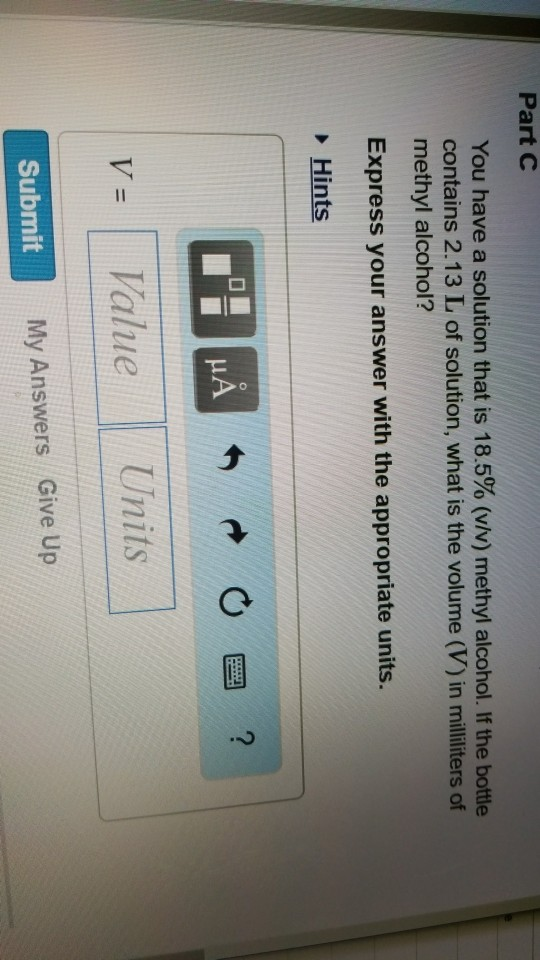Part C You have a solution that is 18.5% (v/v) methyl alcohol. If the bottle contains 2.13 L of solution, what is the volume (V) in milliliters of methyl alcohol? Express your answer with the appropriate units Hints 떠 Submit My Answers Give Up

• ### 30. If we wish to prepare 250 mL of 0.200 M BaCl, solution, how much solid...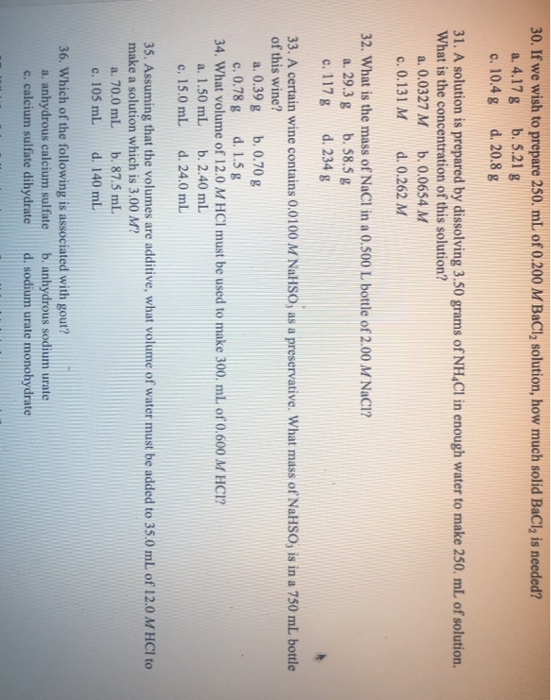30. If we wish to prepare 250 mL of 0.200 M BaCl, solution, how much solid BaCl, is needed? a. 4.17 g b.5.21 g c. 10.4 g d. 20.8 g 31. A solution is prepared by dissolving 3.50 grams of NHCl in enough water to make 250 mL of solution. What is the concentration of this solution? a. 0.0327 M b. 0.0654 M c. 0.131 M d. 0.262 M 32. What is the mass of NaCl in a 0.500 L...

Free Homework App